# Modifying the RLMeta metacompiler

Published on 2019-05-28.

In the first article about RLMeta I wrote that its small implementation makes it feasible to understand and modify. But modifying the RLMeta metacompiler differs from modifying a regular program. In this article I explain how it differs and show examples of how different parts of RLMeta can be modified.

## Meta what?

What is a metacompiler and how does it differ from a regular compiler?

A compiler translates source code into an executable: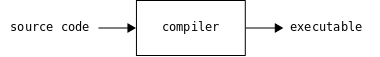A C compiler translates source code written in C into an executable: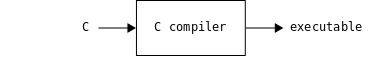A metacompiler translates source code written in a metalanguage into an executable: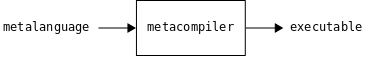A metalanguage is a domain specific language for describing other languages. It can be used to describe what languages look like (their syntax) and how to translate them into executables (their semantics). In essence, it can be used to describe compilers.

Because a metalanguage can be used to describe any compiler, it can also be used to describe the metacompiler itself. The metacompiler translates such a description into itself: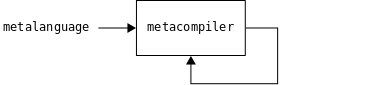A metacompiler does not have to be implemented in the metalanguage itself, but I suspect that it is common that it is. RLMeta is indeed implemented in its own language. This is also known as self-hosting. For the rest of this article, I will assume that a metacompiler is also self-hosting.

A C compiler written in C is not a metacompiler because C is not a metalanguage. It is a general purpose programming language not designed specifically to describe other languages. Such a compiler is merely a self-hosting compiler.

## Modifying a metacompiler

How is modifying a metacompiler different from modifying a regular program?

In general, to modify a program written in a compiled language, its source code and a compiler is needed. Modifying the program is a matter of modifying the source code and compiling it: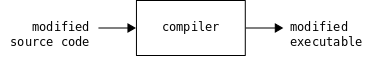In the case of a metacompiler, the compiler and the executable is the same. However, if the source code is modified, the executable might not be the metacompiler itself: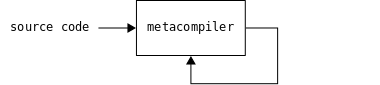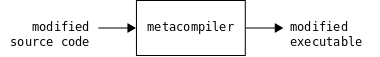At this point, the metacompiler is needed to keep maintaining the modified executable. But the source code for the metacompiler itself is "lost" because the metacompiler can not be reproduced from the modified source code: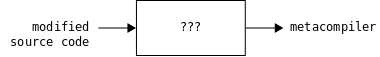Because there is no source code, the original metacompiler can not be modified either.

When modifying a metacompiler, care has to be taken to ensure that, in the end, the executable is still a metacompiler. Otherwise the metacompiler can not be modified further. This is a key difference from modifying a regular program. (Similar care has to be taken when modifying a self-hosting compiler.)

## Compiling RLMeta

This is a recap of how RLMeta is compiled.

RLMeta is compiled with the compile.sh script. It takes one argument which is the path to the RLMeta compiler. The script uses that compiler to compile the source code and then assemble the output to a Python file that looks like this:

1. compile.sh
2. rlmeta template
import sys

SUPPORT = $support_py_string$support_py

$parser_py$codegenerator_py

join = "".join

def compile_grammar(grammar):
parser = Parser()
code_generator = CodeGenerator()
return code_generator.run("ast", parser.run("grammar", grammar))

if __name__ == "__main__":
if "--support" in sys.argv:
sys.stdout.write(SUPPORT)
else:
try:
except _MatchError as e:
sys.stderr.write(e.describe())
sys.exit(1)


The variables $support_py, $parser_py, and $codegenerator_py are produced by the RLMeta compiler. The variable $support_py_string is produced by converting the support library to a Python string. Here is the rest of the compile.sh script:

1. compile.sh
#!/bin/bash

set -e

rlmeta_compiler="$(pwd)/$1"

cd "$(dirname "$0")"

to_python_string() {
}

support_py_string=$(to_python_string < support.py) support_py=$(python "$rlmeta_compiler" --support) parser_py=$(python "$rlmeta_compiler" < parser.rlmeta) codegenerator_py=$(python "$rlmeta_compiler" < codegenerator.rlmeta) cat <<EOF <<rlmeta template>> EOF  ## Verifying metacompiler How can it be verified that RLMeta is a metacompiler? The is_metacompiler.sh script checks if a given file is a metacompiler. It does so by comparing the file to the file generated by the compile.sh script. If they are equal, the file is a metacompiler because it reproduced itself exactly. Otherwise the two files differ. 1. is_metacompiler.sh #!/bin/bash if diff "$1" <(./compile.sh "$1"); then echo "$1 is a metacompiler!"
else
echo "$1 is not a metacompiler. See diff above." fi  RLMeta is a metacompiler because its source code is written in a metalanguage and it is able to reproduce itself: $ ./is_metacompiler.sh rlmeta.py
rlmeta.py is a metacompiler!


The following sections show examples of how to make modifications to RLMeta that ensure that the modified version is still a metacompiler.

## Modifying format of generated code

The code generator in RLMeta generates lambda expressions with newlines and indentation:

(lambda:
...
)


This example shows how to modify it to generate equivalent lambda expressions without the additional punctuation like this:

(lambda: ...)


First, the following line in the code generator is changed from

| astFnBody:x -> { "(lambda:\n" > x < "\n)" }


to

| astFnBody:x -> { "(lambda: " x ")" }


The modified source code describes a metacompiler whose code generator generates lambda expressions without the additional punctuation. How is a new metacompiler created from this modified source code?

Compiling the modified source code gives a new executable:

$./compile.sh rlmeta.py > rlmeta1.py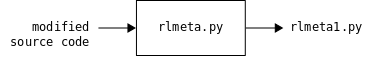With this modification, rlmeta.py is no longer a metacompiler because it differs from rlmeta1.py: $ ./is_metacompiler.sh rlmeta.py
1685,1686c1685
<                                         '(lambda:\n',
<                                         _IndentBuilder(),
---
>                                         '(lambda:',
1688,1689c1687
<                                         _DedentBuilder(),
<                                         '\n)',
---
>                                         ')',
rlmeta.py is not a metacompiler. See diff above.


The difference is that the code for generating lambda expressions is different. rlmeta1.py should still be a compiler that recognizes the same source language so it can be used to compile the modified source code again, giving yet another executable:

$./compile.sh rlmeta1.py > rlmeta2.py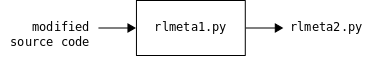rlmeta1.py is not a metacompiler either because it differs from rlmeta2.py: $ ./is_metacompiler.sh rlmeta1.py
287,325c287,299
<         return (lambda:
<             self._or([
<                 (lambda:
<                     (lambda _vars:
<                         (lambda:
<                             self._and([
<                                 (lambda:
<                                     _vars.bind('x', (lambda:
<                                         self._match_rule('name')
<                                     )())
<                                 ),
<                                 (lambda:
<                                     self._match_rule('space')
<                                 ),
<                                 (lambda:
<                                     self._match_charseq('{')
<                                 ),
<                                 (lambda:
<                                     _vars.bind('ys', (lambda:
<                                         self._star((lambda:
<                                             self._match_rule('rule')
<                                         ))
<                                     )())
<                                 ),
<                                 (lambda:
<                                     self._match_rule('space')
<                                 ),
<                                 (lambda:
<                                     self._match_charseq('}')
<                                 ),
<                                 (lambda:
<                                     _SemanticAction(lambda: (['Grammar']+[_vars.lookup('x').eval()]+_vars.lookup('ys').eval()+[]))
<                                 ),
<                             ])
<                         )()
<                     )(_Vars())
<                 ),
<             ])
<         )()
---
>         return (lambda: self._or([
>             (lambda: (lambda _vars:
>                 (lambda: self._and([
>                     (lambda: _vars.bind('x', (lambda: self._match_rule('name'))())),
>                     (lambda: self._match_rule('space')),
>                     (lambda: self._match_charseq('{')),
>                     (lambda: _vars.bind('ys', (lambda: self._star((lambda: self._match_rule('rule'))))())),
>                     (lambda: self._match_rule('space')),
>                     (lambda: self._match_charseq('}')),
>                     (lambda: _SemanticAction(lambda: (['Grammar']+[_vars.lookup('x').eval()]+_vars.lookup('ys').eval()+[]))),
>                 ]))()
>             )(_Vars())),
>         ]))()
...
rlmeta1.py is not a metacompiler. See diff above.


This time the difference is only in formatting. The two programs should be semantically equivalent. The original modification set out to remove additional punctuation in lambda expressions and this can now be seen in the generated code. rlmeta2.py can be used to compile the modified source code again, giving yet another executable:

$./compile.sh rlmeta2.py > rlmeta3.py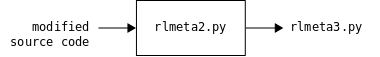rlmeta2.py is now a metacompiler because it is the same as rlmeta3.py: $ ./is_metacompiler.sh rlmeta2.py
rlmeta2.py is a metacompiler!


rlmeta2.py together with the modified source becomes the next version of the RLMeta metacompiler. All other intermediate compilers can be discarded.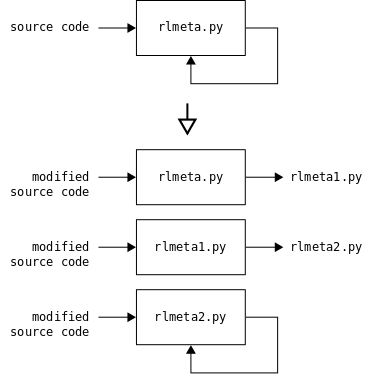## Meta compile script

The steps to create the next version of RLMeta can be scripted like this:

1. meta_compile.sh
#!/bin/bash

set -e

cd "$(dirname "$0")"

./compile.sh rlmeta.py > rlmeta1.py

./compile.sh rlmeta1.py > rlmeta2.py

./compile.sh rlmeta2.py > rlmeta3.py

diff rlmeta2.py rlmeta3.py

diff support.py <(python rlmeta3.py --support)

mv rlmeta3.py rlmeta2.py

mv rlmeta2.py rlmeta1.py

mv rlmeta1.py rlmeta.py

echo OK


It starts with a set -e directive that makes the script stop immediately when a command fails. Then it changes directory to the same directory as the script itself is in. That directory is assumed to also contain the compile.sh script. It then uses the compile.sh script to create the intermediate compilers. If all compile commands succeed, the two last compilers are compared. If they differ, it was not possible to create a metacompiler. The diff command then fails and the script fails. The intermediate compilers are left on disk for inspection. If the two last compilers are the same, a sanity check that the support libraries are the same is done. Then intermediate compilers are removed. At the end, an 'OK' is printed, signifying that a new version of RLMeta was successfully created.

This script will be used in the following examples.

## Breaking modification

This example shows what happens if the source code is modified so that it no longer correctly describes a metacompiler.

A dummy modification that should break things is changing the following line that generates code for rules from

| ["Rule" .:x ast:y] -> { "\ndef _rule_" x "(self):\n" > "return " y "()\n" < }


to

| ["Rule" .:x ast:y] -> { "\ndef _rule_" x "(self):\n" > "pass\n" < }


Instead of generating a function body, the y ast node is ignored and a pass statement is generated instead.

Running the meta compile script on the modified source code indeed gives an error:

$./meta_compile.sh Traceback (most recent call last): File "rlmeta2.py", line 375, in <module> sys.stdout.write(compile_grammar(sys.stdin.read())) File "rlmeta2.py", line 368, in compile_grammar return code_generator.run("ast", parser.run("grammar", grammar)) File "rlmeta2.py", line 102, in run result = self._match_rule(rule_name).eval() AttributeError: 'NoneType' object has no attribute 'eval'  This means that a new metacompiler could not be created. Why? The error happens in rlmeta2.py when the following compilation step is run: ./compile.sh rlmeta2.py > rlmeta3.py  It means that both rlmeta1.py and rlmeta2.py were successfully created, but it failed to create rlmeta3.py. The difference between rlmeta.py and rlmeta1.py is that the code for generating code for rules is different: $ diff rlmeta.py rlmeta1.py
1453,1455c1453
<                                         'return ',
<                                         _vars.lookup('y').eval(),
<                                         '()\n',
---
>                                         'pass\n',


rlmeta1.py is still a compiler that recognizes the same source language so it was successfully used to create rlmeta2.py. The difference between rlmeta1.py and rlmeta2.py is that the code for rules just have a pass statement instead of code for doing matching and returning results:

$diff rlmeta1.py rlmeta2.py 287,325c287 < return (lambda: < self._or([ ... < ]) < )() --- > pass ...  So rlmeta2.py will not work because all rules return None. When _match_rule is called it will get None back but expect a semantic action. When eval is run it will fail because the result is not a semantic action, but instead None. Because the modified source code does not correctly describe how code for rules should be generated, it does not describe a metacompiler, and it can never be used to create a new metacompiler. ## Modifying support library This example shows how to make a modification that requires modifying both the support library and the code generator. It shows how the arguments to the bind function can be swapped. In the support library, the following line that defines the bind function is changed from def bind(self, name, value):  to def bind(self, value, name):  In the code generator, the following line that generates calls to bind is changed from | ["Bind" .:x ast:y] -> { "_vars.bind(" repr(x) ", " y "())" }  to | ["Bind" .:x ast:y] -> { "_vars.bind(" y "(), " repr(x) ")" }  Running the meta compile script on the modified source code gives a new metacompiler: $ ./meta_compile.sh
OK


The difference between the two metacompilers is that the two versions of the support library are modified, all bind calls have swapped arguments, and code that generates calls to bind have swapped arguments:

$diff rlmeta.py.orig rlmeta.py 3c3 < SUPPORT = ... --- > SUPPORT = ... 110c110 < def bind(self, name, value): --- > def bind(self, value, name): 294c294 < _vars.bind('x', (lambda: --- > _vars.bind((lambda: 296c296 < )()) --- > )(), 'x') 1814a1815,1816 > _vars.lookup('y').eval(), > '(), ', 1818,1820c1820 < ', ', < _vars.lookup('y').eval(), < '())', --- > ')', ...  This modification creates a new version of RLMeta that works exactly the same as the previous version, but internally the bind function has a different signature. These compilation steps were not possible in the first version of RLMeta because the compile.sh script generated the support library incorrectly. All RLMeta compilers have two versions of the support library: the one that it generates (stored in the SUPPORT variable), and the one it uses itself. If the compiler is a metacompiler, the two versions are the same, but in intermediate compilers they might differ. The first version of RLMeta generated the support library like this: support_py=$(cat support.py)


It meant that the intermediate compilers always had the latest support library, and the two versions of the support library were always the same.

Running the meta compile script on the modified source code with the old version of compile.sh gives an error:

$./meta_compile.sh Traceback (most recent call last): File "rlmeta1.py", line 2217, in <module> sys.stdout.write(compile_grammar(sys.stdin.read())) File "rlmeta1.py", line 2210, in compile_grammar return code_generator.run("ast", parser.run("grammar", grammar)) File "rlmeta1.py", line 102, in run result = self._match_rule(rule_name).eval() File "rlmeta1.py", line 123, in eval return self.fn() File "rlmeta1.py", line 318, in <lambda> _SemanticAction(lambda: (['Grammar']+[_vars.lookup('x').eval()]+_vars.lookup('ys').eval()+[])) File "rlmeta1.py", line 115, in lookup return self[name] KeyError: 'x'  Now rlmeta1.py has swapped arguments in the support library, but the generated code (generated by rlmeta.py) still expects not swapped arguments. Notice the absence of swapped arguments in bind calls in the diff: $ diff rlmeta.py rlmeta1.py
3c3
< SUPPORT = ...
---
> SUPPORT = ...
110c110
<     def bind(self, name, value):
---
>     def bind(self, value, name):
1814a1815,1816
>                                         _vars.lookup('y').eval(),
>                                         '(), ',
1818,1820c1820
<                                         ', ',
<                                         _vars.lookup('y').eval(),
<                                         '())',
---
>                                         ')',


This in turn leads to rlmeta1.py not functioning because its bind calls have not stored the name as key, but the value. Hence the key error.

The version of RLMeta in this article fixes the generation of the $support_py variable like this: support_py=$(python "$rlmeta_compiler" --support)  This ensures that the code that the code generator generates is always in sync with the support library. ## Extending parser escape codes The escape codes possible in strings in RLMeta are '\\', '\'', '\"', and '\n': escape = '\\' -> "\\" | '\'' -> "'" | '"' -> "\"" | 'n' -> "\n"  This example shows how a new escape code, '\t', can be added. A first attempt might be to extend the escape rule in the parser like this: escape = '\\' -> "\\" | '\'' -> "'" | '"' -> "\"" | 'n' -> "\n" | 't' -> "\t"  Running the meta compile script on the modified source code gives a new metacompiler: $ ./meta_compile.sh
OK


The code generated for the tab case looks like this:

(lambda:
(lambda _vars:
(lambda:
self._and([
(lambda:
self._match_charseq('t')
),
(lambda:
_SemanticAction(lambda: '\\t')
),
])
)()
)(_Vars())
),


It doesn't look quite right. The semantic action does not return a tab character but rather two characters: '\' and 't'. The reason for this is that the modified source code tries to use the new escape code before the parser understands it. Since the parser does not recognize the new escape code, it will treat it as two separate characters.

How can the semantic action be modified to return an single tab character? The escape code can not be used until it is implemented, so the only solution is to call a function that returns a tab character:

escape = '\\' -> "\\" | '\'' -> "'"
| '"'  -> "\"" | 'n'  -> "\n"
| 't'  -> tab()


The tab function is added to the Python template to give generated code access to it:

tab = lambda: "\t"


Running the meta compile script on the modified source code gives a new metacompiler:

$./meta_compile.sh OK  The code generated for the tab case now looks like this: (lambda: (lambda _vars: (lambda: self._and([ (lambda: self._match_charseq('t') ), (lambda: _SemanticAction(lambda: tab( )) ), ]) )() )(_Vars()) ),  The semantic action now correctly generates a tab character by calling the tab function. Now the new escape code can be used inside strings and the tab function can be discarded: escape = '\\' -> "\\" | '\'' -> "'" | '"' -> "\"" | 'n' -> "\n" | 't' -> "\t"  Running the meta compile script on the modified source code gives a new metacompiler: $ ./meta_compile.sh
OK


The code generated for the tab case now looks like this:

(lambda:
(lambda _vars:
(lambda:
self._and([
(lambda:
self._match_charseq('t')
),
(lambda:
_SemanticAction(lambda: '\t')
),
])
)()
)(_Vars())
),


The semantic action now returns a single tab character without using the tab function.

Adding the tab escape code required two passes: one to recognize it and one to use it.

## Modifying parser

The syntax for introducing a semantic action in RLMeta is ->. This example shows how it can be changed to =>. A first attempt might be to change the following line in the parser from

| space '->' hostExpr:x -> ["SemanticAction" x]


to

| space '=>' hostExpr:x -> ["SemanticAction" x]


Running the meta compile script on the modified source code gives an error:

$./meta_compile.sh ... ERROR: L003:C048: expected '}' but found '-'  What happened? The first compiler generated by the meta compile script (rlmeta1.py) has a parser that expects the syntax to be => for semantic actions. When the meta compile script tries to run that compiler on the modified source code it fails because the modified source code is still using the old -> syntax. The source code can not be changed to the new syntax before the parser recognizes it, so the meta compile script does not work for this modification. This can be solved in two ways. Either the parser can be changed to allow both syntaxes like this: | space ('-'|'=')'>' hostExpr:x -> ["SemanticAction" x]  Once a new metacompiler has been created, the source code can be changed to use =>, and then the choice in the parser can be removed to allow only =>. Or the source code must be changed again before creating rlmeta2.py like this: $ # make parser change
$./compile.sh rlmeta.py > rlmeta1.py$ # change syntax from -> to =>
$./compile.sh rlmeta1.py > rlmeta2.py$ ./is_metacompiler.sh rlmeta2.py
rlmeta2.py is a metacompiler!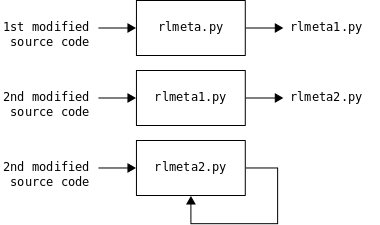## Code listings for RLMeta

The only modifications from the original RLMeta are the tweaked compile.sh script and the additional meta_compile.sh and is_metacompiler.sh scripts. They are shown fully here. For the rest of the source code, see the original article.

### compile.sh

#!/bin/bash

set -e

rlmeta_compiler="$(pwd)/$1"

cd "$(dirname "$0")"

to_python_string() {
support_py_string=$(to_python_string < support.py) support_py=$(python "$rlmeta_compiler" --support) parser_py=$(python "$rlmeta_compiler" < parser.rlmeta) codegenerator_py=$(python "$rlmeta_compiler" < codegenerator.rlmeta) cat <<EOF import sys SUPPORT =$support_py_string
$support_py$parser_py